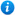# Adding and Subtracting Fractions - Year 4

Adding and Subtracting Fractions - Year 4

Year 4 maths programme of study - Number - fractions (including decimals)

• add and subtract fractions with the same denominator

'Adding and Subtracting Fractions - Year 4' is a KS2 maths teaching resource ideal to use in a lesson covering the year 4 curriculum objective listed above. This colourful and engaging PowerPoint presentation builds on previous knowledge learnt to include increasingly complex problems beyond one whole. Content includes:

• What are fractions recap
• Adding fractions with the same denominator explanation
• Adding fractions with the same denominator activity with an accompanying differentiated worksheet
• Adding fractions beyond one explanation
• Adding fractions beyond one activity with an accompanying differentiated worksheet
• Subtracting fractions with the same denominator explanation
• Subtracting fractions with the same denominator activity with an accompanying differentiated worksheet
• Adding and subtracting fractions word problems activity with an accompanying worksheet
• Adding fractions sorting activity with an accompanying worksheet
• 1 further adding fractions with the same denominator differentiated worksheet with answer
• 1 further subtracting fractions with the same denominator differentiated worksheet with answers

'Adding and Subtracting Fractions - Year 4' can be edited so teachers are able to adapt the resource to meet the needs of each class they teach.

'Adding and Subtracting Fractions - Year 4' can be previewed in full by clicking on the images above.

Our Price : £3.50 / 4 Credits